# Millikan Oil Drop Experiment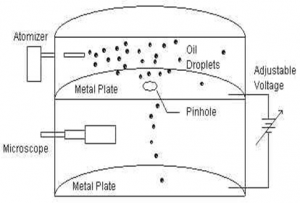The charge of an oil drop can be determined by using Millikan’s oil drop experiment which is ased on th measurement of terminal velocity of oil drop during free fall under gravity and terminal velocity of oil drop under electric field and gravity.

Construction:
In fig P1 and P2 are two electric plate to establish electric field of strength E. Atomiser helps to drop fine drop of oil betwn parallel plates. Microscope helps to study the motion of oil drop. Throughout the experiment the temp is maintained constant and in helps to maintain the required potential.

Working principle:
When oil drop moves between the plates it get charged, let oil drop moves under gravity having free fall then it remains under the action of two different forces ; force due to gravity (which acts vertically downward) and up thrust provided by air particle (which acts in upward direction)
If the oil drop moves with constant velocity (Vg) also called terminal velocity then the net downward force is,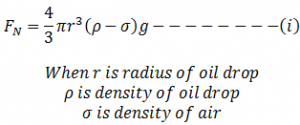Now, according to Stoke’s law as the oil drop moves downward, viscous force (backward direction) is developed which is given by: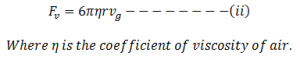Then, in equilibrium,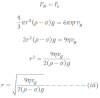This gives the radius of oil drop.

Now if electric field is applied, usually the negatively charged oil drop moves towards the positive plate. If the oil drop moves with a constant velocity ve .Then the force due to electric field is equal to net downward force.

Mathematically, it can be expressed as: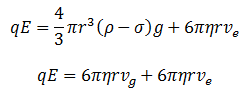At equilibrium condition equation 1 and 2 are equal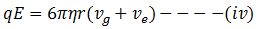Now putting the value of r in equation (iv) we get,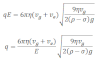This expression gives the charge of an oil drop. In millikan’s oil drop experiment the value of q can be determined if the values of symbols are known.